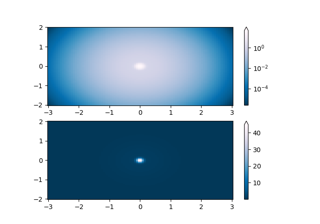# matplotlib.colors.TwoSlopeNorm#

class matplotlib.colors.TwoSlopeNorm(vcenter, vmin=None, vmax=None)[source]#

Bases: `Normalize`

Normalize data with a set center.

Useful when mapping data with an unequal rates of change around a conceptual center, e.g., data that range from -2 to 4, with 0 as the midpoint.

Parameters:
vcenterfloat

The data value that defines `0.5` in the normalization.

vminfloat, optional

The data value that defines `0.0` in the normalization. Defaults to the min value of the dataset.

vmaxfloat, optional

The data value that defines `1.0` in the normalization. Defaults to the max value of the dataset.

Examples

This maps data value -4000 to 0., 0 to 0.5, and +10000 to 1.0; data between is linearly interpolated:

```>>> import matplotlib.colors as mcolors
>>> offset = mcolors.TwoSlopeNorm(vmin=-4000.,
vcenter=0., vmax=10000)
>>> data = [-4000., -2000., 0., 2500., 5000., 7500., 10000.]
>>> offset(data)
array([0., 0.25, 0.5, 0.625, 0.75, 0.875, 1.0])
```
__call__(value, clip=None)[source]#

Map value to the interval [0, 1]. The clip argument is unused.

autoscale_None(A)[source]#

Get vmin and vmax, and then clip at vcenter

inverse(value)[source]#
property vcenter#

## Examples using `matplotlib.colors.TwoSlopeNorm`#Colormap Normalization

Colormap Normalization